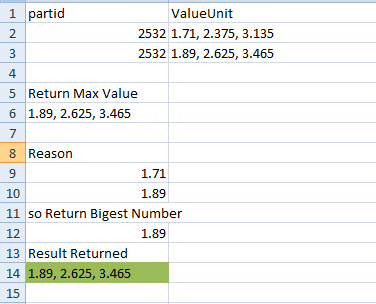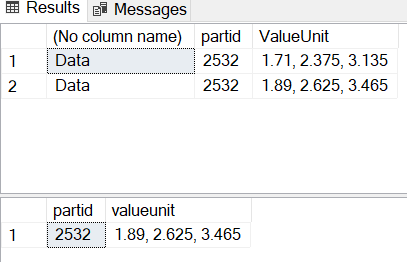# How to get max Value Unit from field Value Unit based on first value before comma separated?

I work on SQL server 2012 I face issue :i can't get first max value from value Unit based on First value before comma .

as example This value Unit below :

```````1.89, 2.625, 3.465`
``````

I will get first value before comma separated as 1.89 then if this number is max value return full number
exist on Value Unit

``````    create table #finaltable
(
partid  int,
ValueUnit nvarchar(50)
)
insert into #finaltable(partid,ValueUnit)
values
(2532,'1.71, 2.375, 3.135'),
(2532,'1.89, 2.625, 3.465')
select * from #finaltable
``````

How to get first max value from field ValueUnit based on first value before comma separated ?

Expected Result returned :

` 1.89, 2.625, 3.465`
because 1.89 is maximum number from 1.71 then I returned full number

see image below for clearMaybe:

``````WITH UnitOrder
AS
(
SELECT T.partid, T.ValueUnit
,ROW_NUMBER() OVER (PARTITION BY partid ORDER BY X.FirstValue DESC, T.ValueUnit DESC) AS rn
FROM #finaltable T
CROSS APPLY (VALUES(CAST(LEFT(T.ValueUnit, CHARINDEX(',', T.ValueUnit) -1) AS float))) X (FirstValue)
)
SELECT partid, ValueUnit
FROM UnitOrder
WHERE rn = 1;``````

or, if the first number is always less than 10:

``````WITH UnitOrder
AS
(
SELECT T.partid, T.ValueUnit
,ROW_NUMBER() OVER (PARTITION BY partid ORDER BY T.ValueUnit DESC) AS rn
FROM #finaltable T
)
SELECT partid, ValueUnit
FROM UnitOrder
WHERE rn = 1;``````
2 Likes

hi

i tried to do this .. Its a different way of doing it ..

if we get into performance tuning .. logical reads .. network round trips .. index seek and index scans and all that ..explain plan ..

then lot of stuff ...
Please let me know if this works for you !!

``````select   a.partid
, valueunit
from
finaltable a
join
(select partid,max(cast(left(valueunit,patindex('%,%',valueunit)-1) as float)) as leftunit from finaltable group by partid) b
on
left(a.valueunit,patindex('%,%',a.valueunit)-1) = b.leftunit
``````1 Like